# The r3PG R package

#### 2023-07-04

Abstract

This vignette provides an overview of the r3PG R package functions and options. We provide a working examples that (i) demonstrates the basic functionality and use of the package, (ii) performs a sensitivity analysis and a Bayesian calibration of the model, and (iii) uses the calibrated model to simulate spatial patterns of forest growth across Switzerland.

## Purpose

r3PG provides an implementation of the Physiological Processes Predicting Growth (3-PG) model (Landsberg & Waring, 1997). The r3PG serves as a flexible and easy-to-use interface for the 3-PGpjs (Sands, 2010) and the 3-PGmix (Forrester & Tang, 2016) models written in Fortran. The package, allows for fast and easy interaction with the model, and Fortran re-implementation facilitates computationally intensive sensitivity analysis and calibration. The user can flexibly switch between various options and submodules to use the original 3-PGpjs model version for monospecific, even-aged and evergreen forests and the 3-PGmix model, which can also simulate multi-cohort stands (e.g. mixtures, uneven-aged) that contain deciduous species.

## Single model runs

To demonstrate the basic functionality of the r3PG R package, we will perform a simple simulation with the 3-PGmix model. The central function to run 3-PG from R is run_3PG. When called, the function will:

• check the model input for the consistency in structure
• replace the default parameters if new ones are provided
• run and return the simulated output from the model.

Before using run_3PG the user must prepare the input data as described in the help of run_3PG. The inputs include information about site conditions, species initial conditions, climate data, and parameters (if they need to be modified).

In this example, we run a simulation for mixed Fagus sylvatica and Pinus sylvestris stands (Forrester et al., 2017). The input data are provided as internal data in r3PG. You can inspect their structure by exploring, e.g., d_site.

library(r3PG)
library(dplyr)
library(ggplot2)

out_3PG <- run_3PG(
site        = d_site,
species     = d_species,
climate     = d_climate,
thinning    = d_thinning,
parameters  = d_parameters,
size_dist   = d_sizeDist,
settings    = list(light_model = 2, transp_model = 2, phys_model = 2,
height_model = 1, correct_bias = 0, calculate_d13c = 0),
check_input = TRUE, df_out = TRUE)

The output of the run_3PG function can be either a long format dataframe or a 4-dimentional array where each row corresponds to one month of simulations, depending on your choice of the variable df_out. If setting df_out = T , the output will be in the array format, where the first dimension is time, the second dimension corresponds to species (each column represents one species), and the third dimension corresponds to variable groups and the variables themselves. In our simulation above, we set df_out = T , which means that our output is in the long format.

head( out_3PG )
#>         date         species   group variable      value
#> 1 2001-01-31 Fagus sylvatica climate  tmp_min -1.4669526
#> 2 2001-02-28 Fagus sylvatica climate  tmp_min -0.8616548
#> 3 2001-03-31 Fagus sylvatica climate  tmp_min  3.8046124
#> 4 2001-04-30 Fagus sylvatica climate  tmp_min  2.7947164
#> 5 2001-05-31 Fagus sylvatica climate  tmp_min  9.7551870
#> 6 2001-06-30 Fagus sylvatica climate  tmp_min 10.0666666

To get information about output variables and their group, please look at data('i_output').

As an example for how to visualize the output, we select six variables: basal area, dbh, height, stem biomass, foliage biomass, and root biomass. For visualization we are using a ggplot function, which allows us to visualize all the outputs side-by-side.

i_var <- c('stems_n',  'dbh', 'height', 'biom_stem', 'biom_root', 'biom_foliage')
i_lab <- c('Stem density', 'DBH', 'Height', 'Stem biomass', 'Root biomass', 'Foliage biomass')

out_3PG %>%
filter(variable %in% i_var) %>%
mutate(variable = factor(variable, levels = i_var)) %>%
ggplot( aes(date, value))+
geom_line( aes(color = species), size = 0.5)+
facet_wrap( ~ variable, scales = 'free_y', ncol = 3,
labeller = labeller(variable = setNames(i_lab, i_var) )) +
scale_color_brewer('', palette = 'Dark2') +
theme_classic()+
theme(legend.position="bottom")+
xlab("Calendar date") + ylab('Value')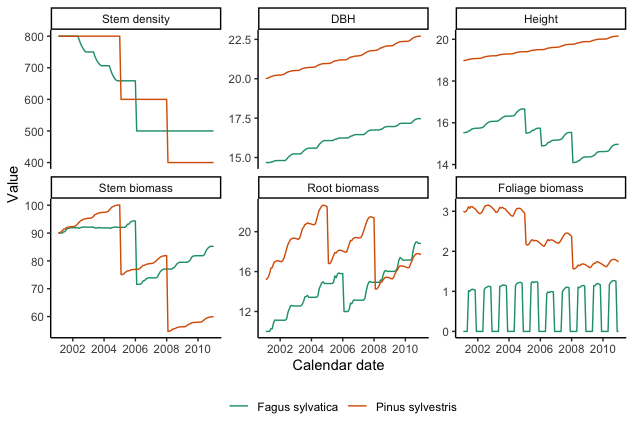## Sensitivity analysis and Bayesian calibration

As a second case study, we want do a slightly more ambitious project, which is a sensitivity analysis and calibration of the model.

For both tasks, we use freely available forest growth data from the PROFOUND database. The data can be extracted from the sql database with the ProfoundData R package, which is available on CRAN. For convenience, however, we have included the extracted and cleaned data in the required format in the r3PG package. All the data used in this vignette can be downloaded from r3PG/vignettes_build

load('vignette_data/solling.rda')

library(tidyr)
library(purrr)
library(BayesianTools)
library(sensitivity)

As a second example, we will first performed a Morris screening and then perform the Bayesian calibration of the 3-PGpjs model. We will use the Solling forest flux site located in Germany at an elevation of 508 m.a.s.l., and dominated by Picea abies. We obtained data for this site via the PROFOUND database that was set up for evaluating vegetation models and simulating climate impacts on forests (Reyer et al., 2019). There are almost 50 years of records for various stand and flux variables for Solling site.

### Likelihood function

A sensitivity analysis requires a target variable for which we want to evaluate sensitivity. In principle, any variable that is predicted by the model could be used as target, including any of the variables that we plotted above. In this case, we are planning to run a Bayesian calibration later, and thus it makes sense to run the sensitivity on the difference between model predictions and data, which is measured by the likelihood function.

For the likelihood, we assumed normal errors for the six variables that describe stand stocks and characteristics: basal area, dbh, height, stem biomass, foliage biomass, and root biomass. To calculate the stand-level stocks, we applied the biomass equations developed for European forests following Forrester et al. (2017) for each measured tree, and summed it up to the stand level in Mg dry matter $$ha^{-1}$$.

r3pg_sim <- function( par_df, ...){
#' @description function to simulate the model for a given parameter
#' @param par_df data frame of the parameters with two columns: parameter, piab

sim.df <- run_3PG(
site = site_solling,
species = species_solling,
climate = climate_solling,
thinning = thinn_solling,
parameters = par_df,
size_dist = NULL,
settings =  list(light_model = 1, transp_model = 1, phys_model = 1),
check_input = TRUE, ...)

return( sim.df )
}

r3pg_ll <- function( par_v ){
#' @param par_v a vector of parameters for the calibration, including errors
#' par_v = par_cal_best

# replace the default values for the selected parameters
err_id = grep('err', par_cal_names)

par_df = dplyr::bind_rows(
dplyr::filter(par_def.df, !parameter %in% par_cal_names),
data.frame(parameter = par_cal_names[-err_id], piab = par_v[-err_id]))

err_v = par_v[err_id]

# simulate the model
sim.df <- r3pg_sim(par_df, df_out = FALSE)
sim.df <- cbind(sim.df[,,2,c(3,5,6)], sim.df[,,4,c(1,2,3)])

# calculate the log likelihood
logpost <- sapply(1:6, function(i) {
likelihoodIidNormal( sim.df[,i], observ_solling_mat[,i], err_v[i] )
}) %>% sum(.)

if(is.nan(logpost) | is.na(logpost) | logpost == 0 ){logpost <- -Inf}

return( logpost )
}

### Morris sensitivity

To evaluate the sensitivity of the likelihood above to changes of the model parameters, we used Morris screening. Morris screening is a so-called global sensitivity method which quantifies parameter sensitivity in defined area of the parameter space. This means that we require to set min / max values for all model parameters, which should be set on sensible ranges. Here, we provide expert-chosen values for all parameters, including the error parameters that are used in the likelihood.

# default parameters for simulations
par_def.df <- select(param_solling, parameter = param_name, piab = default)

# parameters for calibration and their ranges
param_morris.df <- bind_rows(param_solling, error_solling) %>% filter(!is.na(min))
par_cal_names <- param_morris.df$param_name par_cal_min <- param_morris.df$min
par_cal_max <- param_morris.df$max par_cal_best <- param_morris.df$default

r3pg_ll( par_cal_best)
#>  -192.1321

With these ranges and using the settings specified below, we run a Morris screening with 30500 model evaluations (N (length(factors)+1)) and visualize the results. This code ran approximately 4 minutes on our local computer.

morris_setup <- createBayesianSetup(
likelihood = r3pg_ll,
prior = createUniformPrior(par_cal_min, par_cal_max, par_cal_best),
names = par_cal_names)

set.seed(432)
morrisOut <- morris(
model = morris_setup$posterior$density,
factors = par_cal_names,
r = 500,
design = list(type = "oat", levels = 20, grid.jump = 3),
binf = par_cal_min,
bsup = par_cal_max,
scale = TRUE)

# summaries the morris output
morrisOut.df <- data.frame(
parameter = par_cal_names,
mu.star = apply(abs(morrisOut$ee), 2, mean, na.rm = T), sigma = apply(morrisOut$ee, 2, sd, na.rm = T)
) %>%
arrange( mu.star )
load('vignette_data/morris.rda')

We visualize the results of the morris analysis by listing all the parameters and error parameters. A high $$\mu^{*}$$ indicates a factor with an important overall influence on model output; a high $$\alpha$$ indicates either a factor interacting with other factors or a factor whose effects are non-linear.

morrisOut.df %>%
gather(variable, value, -parameter) %>%
ggplot(aes(reorder(parameter, value), value, fill = variable), color = NA)+
geom_bar(position = position_dodge(), stat = 'identity') +
scale_fill_brewer("", labels = c('mu.star' = expression(mu * "*"), 'sigma' = expression(sigma)), palette="Dark2") +
theme_classic() +
theme(
axis.text = element_text(size = 6),
axis.text.x = element_text(angle=90, hjust=1, vjust = 0.5),
axis.title = element_blank(),
legend.position = c(0.05 ,0.95),legend.justification = c(0.05,0.95)
)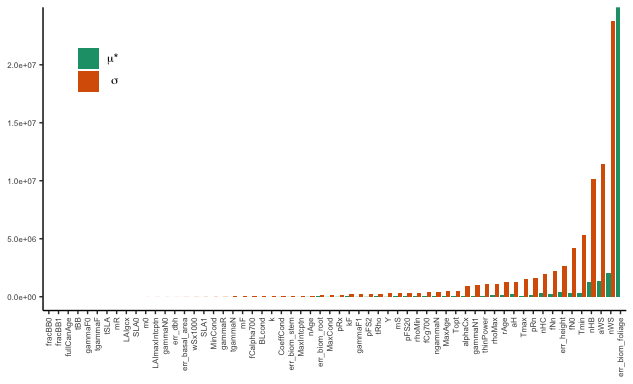### Bayesian calibration

As a next step, we want to calibrate the model. To speed up this task, we calibrate only the 20 most sensitive parameters identified in the morris screening plus the six errors parameters for each of the observational variables.

As Priors for the Bayesian analysis, we used uniform priors with the same ranges that we used for the Morris screening.

To estimate the posterior, we run 3 chains, each for 6e+06 iterations. On a local computer, this takes approximately 21 hours per chain.

# which parameters to calibrate
par_select <- morrisOut.df$parameter %>% .[-grep('err', .)] %>% tail(., 20) %>% as.character() par_id <- which(par_cal_names %in% c(par_select, error_solling$param_name) )

par_cal_names <- par_cal_names[par_id]
par_cal_min <- par_cal_min[par_id]
par_cal_max <- par_cal_max[par_id]
par_cal_best <- par_cal_best[par_id]

mcmc_setup <- createBayesianSetup(
likelihood = r3pg_ll,
prior = createUniformPrior(par_cal_min, par_cal_max, par_cal_best),
names = par_cal_names)
mcmc_out <- runMCMC(
bayesianSetup = mcmc_setup,
sampler = "DEzs",
settings = list(iterations = 6000000, nrChains = 3, thin = 10))
load('vignette_data/mcmc.rda')

As the first step, we can look at the trace plots of the MCMC to estimate the burn-in time. Here, we plot only the first parameters for reasons of space.

plot(mcmc_out, which = c(1,2))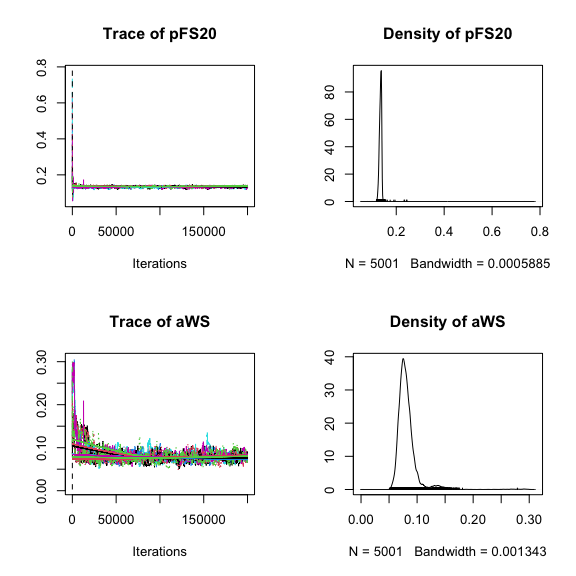The plots suggest that the MCMC has run into equilibrium after approximately 100,000 steps. We can then check convergence of the MCMC for sampling after the burnin. For an MCMC chain to be converged, one typically requires the univariate psrf to be < 1.05 and the multivariate psrf $$\le$$ 1.1 or 1.2, which is the case here.

gelmanDiagnostics(mcmc_out, start =  100000 )
#> Potential scale reduction factors:
#>
#>                  Point est. Upper C.I.
#> pFS20                  1.04       1.08
#> aWS                    1.02       1.04
#> nWS                    1.02       1.04
#> pRn                    1.05       1.11
#> Tmin                   1.03       1.06
#> Topt                   1.03       1.07
#> Tmax                   1.01       1.02
#> fN0                    1.02       1.05
#> fNn                    1.11       1.23
#> MaxAge                 1.02       1.05
#> rAge                   1.04       1.09
#> gammaN1                1.02       1.05
#> thinPower              1.01       1.03
#> mS                     1.02       1.05
#> alphaCx                1.02       1.04
#> rhoMin                 1.01       1.01
#> rhoMax                 1.01       1.03
#> aH                     1.01       1.03
#> nHB                    1.02       1.04
#> nHC                    1.02       1.04
#> err_basal_area         1.09       1.15
#> err_dbh                1.03       1.07
#> err_height             1.01       1.02
#> err_biom_stem          1.01       1.03
#> err_biom_root          1.01       1.02
#> err_biom_foliage       1.01       1.03
#>
#> Multivariate psrf
#>
#> 1.18

After having confirmed that the MCMC is converged, we can summarize the MCMC chains, for example using the summary() or MAP() functions (MAP = maximum aposteriori value = parameter value with the highest posterior probability density). For reasons of space, we do not show output of these functions here.

summary(mcmc_out, start = 100000)
MAP(mcmc_out)

To create model predictions with their uncertainties and evaluate the model performance, we forwarded the parameter uncertainty to model outputs (posterior predictions) by drawing 500 samples from the mcmc object and run model simulations for each of the parameter combinations.

par_def.df <- select(param_solling, parameter = param_name, piab = default)

param.draw <- getSample(mcmc_out, start = 100000, numSamples = 500, coda = F, whichParameters = 1:20) %>%
as.data.frame() %>%
mutate(mcmc_id = 1:n()) %>%
nest_legacy(-mcmc_id, .key = 'pars')   %>%
mutate(
pars = map(pars, unlist),
pars = map(pars, ~tibble::enframe(.x, 'parameter', 'piab')),
pars = map(pars, ~bind_rows(.x, filter(par_def.df, !parameter %in% par_cal_names))))

We then simulate forest growth using the defaults and the drawn parameters combinations. Afterwards, we visualize the posteriori prediction range by drawing the $$5^{th}$$ and $$95^{th}$$ range of predictions. The calibrated model simulates the observational data well.

# default run
def_run.df <- r3pg_sim( par_df = par_def.df, df_out = T) %>%
select(date, variable, value) %>%
mutate(run = 'default')

# calibrated run
post_run.df <- param.draw   %>%
mutate( sim = map( pars, ~r3pg_sim(.x, df_out = T)) ) %>%
select(mcmc_id, sim) %>%
unnest_legacy() %>%
group_by(date, variable) %>%
summarise(
q05 = quantile(value, 0.05, na.rm = T),
q95 = quantile(value, 0.95, na.rm = T),
value = quantile(value, 0.5, na.rm = T)) %>%
ungroup() %>%
mutate(run = 'calibrated')

sim.df <- bind_rows( def_run.df, post_run.df)

# Visualize
i_var <- c('basal_area',  'dbh', 'height', 'biom_stem', 'biom_root', 'biom_foliage')
i_lab <- c('Basal area', 'DBH', 'Height', 'Stem biomass', 'Root biomass', 'Foliage biomass')

observ_solling.df <- observ_solling %>%
gather(variable, value, -date) %>%
filter(variable %in% i_var) %>%
filter(!is.na(value)) %>%
mutate(variable = factor(variable, levels = i_var))

sim.df %>%
filter(variable %in% i_var) %>%
mutate(variable = factor(variable, levels = i_var)) %>%
ggplot(aes(date, value))+
geom_ribbon(aes(ymin = q05, ymax = q95, fill = run), alpha = 0.5)+
geom_line( aes(color = run), size = 0.2)+
geom_point( data = observ_solling.df, color = 'grey10', size = 0.1) +
facet_wrap( ~ variable, scales = 'free_y', nrow = 2, labeller = labeller(variable = setNames(i_lab, i_var) )) +
scale_color_manual('', values = c('calibrated' = '#1b9e77', 'default' = '#d95f02')) +
scale_fill_manual('', values = c('calibrated' = '#1b9e77', 'default' = '#d95f02'), guide = F) +
theme_classic() +
theme(legend.position="bottom")+
xlab("Calendar date") + ylab('Value')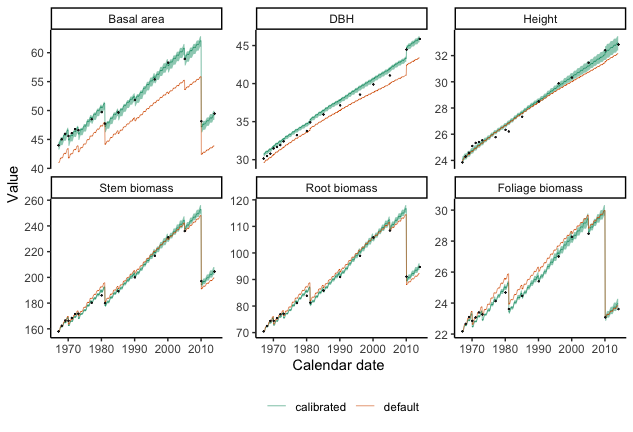## Spatial simulations

3-PG is a cohort level model. Thus, to make a simulation across a landscapes, we need to explicitly simulate each individual grid point. For this purpose, we will simulate Picea abies stand biomass across Switzerland on a 1x1 km grid. In total, this sums to ~18’000 grid points over Switzerland.

We have prepared the input data, including climate and soil information for each of the grid points. We used interpolated meteorological data from the Landscape Dynamics group (WSL, Switzerland) based on data from MeteoSwiss stations (Swiss Federal Office of Meteorology and Climatology) by employing the DAYMET method (Thornton, Running, & White, 1997). Grid-specific information on soil type and plant available soil water was retrieved from European Soil Database Derived data (Hiederer 2013). In addition, we use ~500 samples drawn from the previously obtained mcmc object and run model simulations for each of the parameter combinations to understand simulation uncertainties.

load('vignette_data/grid_input.rda')

We construct a function, which requires site and climate information as input, and calculates the $$5^{th}$$, $$50^{th}$$ and $$95^{th}$$ percentile from ~500 simulated runs. To do so, we use the multidplyr package for distributing computing. In total, it takes less than 1 hour on a computer cluster with 50 processes (CPU time ~20 hours).

library(multidplyr)

r3pg_grid <- function(site, forc){
#' @description simulate the n runs for a given site with the drawn parameter combination

r3pg_int <- function( par_df){
#' @description function to run one site and return required output on standing biomass
#' @param par_df a data.frame of parameters

out <- run_3PG(site, species.grid, forc, thinn.grid, par_df, NULL,
list(light_model = 1, transp_model = 1, phys_model = 1), check_input = TRUE,
df_out = F)[,,4,1]

return( last( out ) )
}

site_out <- param.draw %>%
mutate( sim = map( pars, r3pg_int)) %>%
select(mcmc_id, sim) %>%
unnest_legacy() %>%
summarise(
q05 = quantile(sim, 0.05, na.rm = T),
q95 = quantile(sim, 0.95, na.rm = T),
value = quantile(sim, 0.5, na.rm = T))

return( site_out )
}

cl_in <- new_cluster(n = 2) %>% # or more cores if desired
cluster_library(c('r3PG', 'purrr', 'dplyr', 'tidyr')) %>%
cluster_copy(names = c("r3pg_grid", "species.grid", "thinn.grid", "param.draw"))

#' hide the sample_n
sim.grid <- inner_join(site.grid, climate.grid, by = 'grid_id')  %>%
sample_n(10) %>% # remove this for full simulation
partition(cluster = cl_in) %>%
mutate( out = map2(site, forc, ~r3pg_grid(.x, .y))) %>%
select( grid_id, out) %>%
collect() %>%
unnest_legacy() %>%
ungroup()
load('vignette_data/grid_sim.rda')

Once the simulations are done, we visualize the results for average standing biomass and associated uncertainties for the whole landscape.

sim.grid %>%
mutate( range = q95 - q05) %>%
select( grid_id, mean = value, range) %>%
gather( variable, value, -grid_id) %>%
inner_join( ., coord.grid, by = 'grid_id') %>%
ggplot( aes(x, y, fill = value) ) +
geom_raster()+
facet_wrap(~variable)+
theme_void()+
coord_equal() +
scale_fill_distiller( '', palette = 'Spectral', limits = c(0, 250))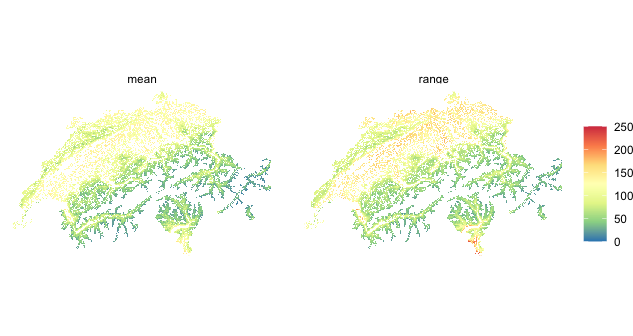## Session

sessionInfo()
#> R version 4.2.0 (2022-04-22)
#> Platform: x86_64-apple-darwin17.0 (64-bit)
#> Running under: macOS Big Sur/Monterey 10.16
#>
#> Matrix products: default
#> BLAS:   /Library/Frameworks/R.framework/Versions/4.2/Resources/lib/libRblas.0.dylib
#> LAPACK: /Library/Frameworks/R.framework/Versions/4.2/Resources/lib/libRlapack.dylib
#>
#> locale:
#>  en_US.UTF-8/en_US.UTF-8/en_US.UTF-8/C/en_US.UTF-8/en_US.UTF-8
#>
#> attached base packages:
#>  stats     graphics  grDevices utils     datasets  methods   base
#>
#> other attached packages:
#>  sensitivity_1.27.0  BayesianTools_0.1.7 purrr_0.3.4
#>  tidyr_1.2.0         ggplot2_3.3.6       dplyr_1.0.8
#>  r3PG_0.1.5          knitr_1.38
#>
#> loaded via a namespace (and not attached):
#>   Brobdingnag_1.2-7    tidyselect_1.1.2     xfun_0.30
#>   bslib_0.3.1          splines_4.2.0        lattice_0.20-45
#>   colorspace_2.0-3     vctrs_0.4.1          generics_0.1.2
#>  htmltools_0.5.2      yaml_2.3.5           utf8_1.2.2
#>  rlang_1.0.6          nloptr_2.0.1         jquerylib_0.1.4
#>  pillar_1.7.0         glue_1.6.2           withr_2.5.0
#>  DBI_1.1.2            RColorBrewer_1.1-3   foreach_1.5.2
#>  lifecycle_1.0.1      stringr_1.4.0        munsell_0.5.0
#>  gtable_0.3.0         mvtnorm_1.1-3        codetools_0.2-18
#>  coda_0.19-4          evaluate_0.15        labeling_0.4.2
#>  fastmap_1.1.0        numbers_0.8-2        fansi_1.0.3
#>  highr_0.9            Rcpp_1.0.8.3         scales_1.2.0
#>  DHARMa_0.4.5         jsonlite_1.8.0       farver_2.1.0
#>  lme4_1.1-29          digest_0.6.29        stringi_1.7.6
#>  grid_4.2.0           cli_3.4.1            tools_4.2.0
#>  magrittr_2.0.3       sass_0.4.1           tibble_3.1.7
#>  crayon_1.5.1         pkgconfig_2.0.3      ellipsis_0.3.2
#>  MASS_7.3-56          Matrix_1.4-1         iterators_1.0.14
#>  bridgesampling_1.1-2 assertthat_0.2.1     minqa_1.2.4
#>  rmarkdown_2.14       rstudioapi_0.13      R6_2.5.1
#>  boot_1.3-28          nlme_3.1-157         compiler_4.2.0